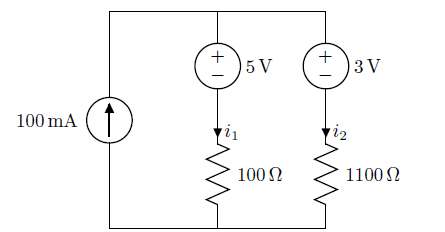# Circuit with 2 voltage sources and 1 current source

• Engineering
• ichabodgrant

#### ichabodgrantHere is the circuit. I have to find the voltage across each resistor and the current i1 and i2.
My attempts are the following:

By KCL,
i2 + i1 = 0.1 A

By KVL,
3 - 5 - 100i1 + 1100i2 = 0

∴ i1 = 0.09 A, i2 = 0.01A
∴ Voltage across 100Ω resistor = 0.09 × 100 = 9 V, voltage across 1100Ω resistor = 0.01 × 1100 = 11 V

Are there any mistakes?

Last edited:

•